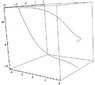# How to show integral equal to hypergeometric function?

• jackmell

#### jackmell

Hi,

I would like to show directly,

$$\int \frac{e^{at}}{e^{it}+e^{-it}}dt=\frac{e^{(i+a) t} \text{Hypergeometric2F1}\left[1,\frac{1}{2}-\frac{i a}{2},\frac{3}{2}-\frac{i a}{2},-e^{2 i t}\right]}{i+a}$$

I realize I can differentiate the antiderivative to show the relation but was wondering how to integrate the integral directly to obtain the hypergeometric function or are these type integrals evaluated using a different technique? I can express it as:

$$\int \frac{e^{(i-a)t}}{1+e^{2it}}dt$$

and at least it has the $e^{2it}$ in there.

How are any integrals expressed in terms of hypergeometric functions?

How are you defining the "Hypergeometric" functions?

(And that last integral should be
$$\int\frac{e^{i-a)t}}{1+ e^{-2it}}dt$$)

(And that last integral should be
$$\int\frac{e^{(i-a)t}}{1+ e^{-2it}}dt$$)
Even that's not right. It should either be
$$\int \frac{e^{(a+i)t}}{1+e^{2it}}dt$$
or
$$\int \frac{e^{(a-i)t}}{1+e^{-2it}}dt$$

Hi,

I would like to show directly,

$$\int \frac{e^{at}}{e^{it}+e^{-it}}dt=\frac{e^{(i+a) t} \text{Hypergeometric2F1}\left[1,\frac{1}{2}-\frac{i a}{2},\frac{3}{2}-\frac{i a}{2},-e^{2 i t}\right]}{i+a}$$

I realize I can differentiate the antiderivative to show the relation but was wondering how to integrate the integral directly to obtain the hypergeometric function or are these type integrals evaluated using a different technique? I can express it as:

$$\int \frac{e^{(i-a)t}}{1+e^{2it}}dt$$

and at least it has the $e^{2it}$ in there.

How are any integrals expressed in terms of hypergeometric functions?
I'd start by trying a substitution, but I honestly don't know how one goes about integrating that directly.

Have you considered approaching it by the hypergeometric differential equation?

How are you defining the "Hypergeometric" functions?

Hi,

I'm defining the hypergeometric function as the analytic continuation of the hypergeometric power series:

$$\mathstrut_2 F_1\left(a,b,c,z\right)=\sum_{n=0}^{\infty} \frac{(a)_n (b)_n}{(c)_n} \frac{z^n}{n!}\quad |z|<1$$

which I believe is a multi-valued function with a branch-point at z=1 but I'm not very familiar with this function.

Have you considered approaching it by the hypergeometric differential equation?

I've considered that but don't know how to approach this integral through that DE but I'll keep working on it.

Also, thanks for correcting the integral DH. It is:

$$\int \frac{e^{(i+a) t}}{1+e^{2it}}dt$$

Last edited:
Hi,

I'm defining the hypergeometric function as the analytic continuation of the hypergeometric power series:

$$\mathstrut_2 F_1\left(a,b,c,z\right)=\sum_{n=0}^{\infty} \frac{(a)_n (b)_n}{(c)_n} \frac{z^n}{n!}\quad |z|<1$$

which I believe is a multi-valued function with a branch-point at z=1 but I'm not very familiar with this function.
Why would it be multivalued? It isn't readily apparent to me.

As to the problem, this is new to me, too. I'll probably piggy-back off of this thread for my own learning. :tongue:

Why would it be multivalued? It isn't readily apparent to me.

As to the problem, this is new to me, too. I'll probably piggy-back off of this thread for my own learning. :tongue:

That's an interesting question in itself. Well, Mathematica says it is although I realize that's not sufficient. However, we can experimentally verify it's multivalued by considering the hypergeometric DE:

$$z(1-z)w''+(c-(a+b+1)z)w'-abw=0$$

and numerically integrating this equation over a contour which encircles the branch point at z=1, for example over a circle of radius 2, that is, by letting $z=2e^{it}$. Now when I do that and substitute that into the DE and I did it quickly so not confident I did it error-free, I obtain the DE in terms of t:

$$z(1-z)(-1/4 e^{-2it} w''-iw')+(c-(a+b+1)z)(-i/2 e^{-it}) w'-ab w=0,\quad z=2e^{it}$$

now, I just arbitrarily let a=1, b=2, c=3, w(0)=2, w'(0)=1 and integrate over 2pi and plot the real (or imaginary) results, the solution does not return to the same point. That is experimentally, a clear sign it's not single-valued although there is always the chance of numerical error.

Also, getting back to the problem, I'm encouraged by my initial attempts at iterative integration by parts, the solution looks like it's getting there but not quite. I'll work on that approach.

#### Attachments

•multivalued hypergeometric function.jpg
11.2 KB · Views: 498
Last edited: